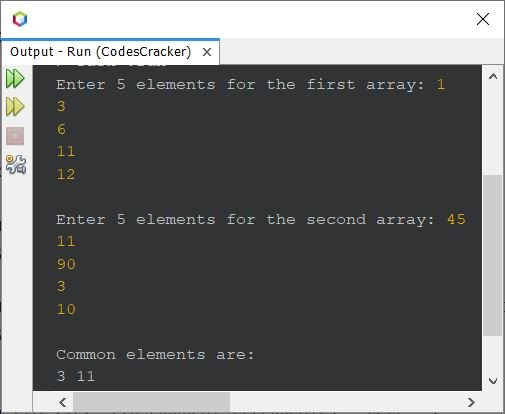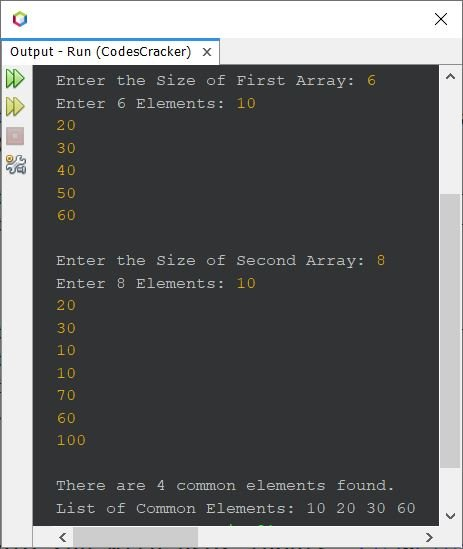# Java Program to Find Common Elements between Two Arrays

This article covers a program in Java that find and prints common elements between two given arrays.

## Find Common Elements between Two Arrays - Basic Version

The question is, write a Java program to find and print common elements available between two arrays. The array must be received by user at run-time of the program. The program given below is the answer to this question:

```import java.util.Scanner;

public class CodesCracker
{
public static void main(String[] args)
{
int[] arrOne = new int;
int[] arrTwo = new int;
int i, j;
Scanner s = new Scanner(System.in);

System.out.print("Enter 5 elements for the first array: ");
for(i=0; i<5; i++)
arrOne[i] = s.nextInt();
System.out.print("\nEnter 5 elements for the second array: ");
for(i=0; i<5; i++)
arrTwo[i] = s.nextInt();

System.out.println("\nCommon elements are:");
for(i=0; i<5; i++)
{
for(j=0; j<5; j++)
{
if(arrOne[i]==arrTwo[j])
System.out.print(arrOne[i]+ " ");
}
}
}
}```

The snapshot given below shows the sample run of above Java program with user inputs, 1, 3, 6, 11, 12 as five elements of first, and 45, 11, 90, 3, 10 as five elements of second array:## Find Common Elements between Two Arrays - Complete Version

There are some limitations with above program, like what if user wants to enter more elements ?
what there is no common elements available in both arrays ?
what if a single common elements available multiple times in both arrays ?
Also the program given above, does not actually stores the common elements, rather it just prints the element at the time of comparing.

And if there is no common elements found in both arrays, then the message Common elements are: will get displayed with no elements on output, that will look weird. Therefore let me modify the above program, keeping in mind, to remove all the limitations:

```import java.util.Scanner;

public class CodesCracker
{
public static void main(String[] args)
{
int i, j, arrOneSize, arrTwoSize, arrCommonSize, k=0, x, check;
Scanner s = new Scanner(System.in);

System.out.print("Enter the Size of First Array: ");
arrOneSize = s.nextInt();
int[] arrOne = new int[arrOneSize];
System.out.print("Enter " +arrOneSize+ " Elements: ");
for(i=0; i<arrOneSize; i++)
arrOne[i] = s.nextInt();

System.out.print("\nEnter the Size of Second Array: ");
arrTwoSize = s.nextInt();
int[] arrTwo = new int[arrTwoSize];
System.out.print("Enter " +arrTwoSize+ " Elements: ");
for(i=0; i<arrTwoSize; i++)
arrTwo[i] = s.nextInt();

if(arrOneSize<arrTwoSize)
arrCommonSize = arrOneSize;
else
arrCommonSize = arrTwoSize;
int[] arrCommon = new int[arrCommonSize];

for(i=0; i<arrOneSize; i++)
{
for(j=0; j<arrTwoSize; j++)
{
if(arrOne[i]==arrTwo[j])
{
check = 0;
for(x=0; x<k; x++)
{
if(arrCommon[x]==arrOne[i])
{
check = 1;
break;
}
}
if(check==0)
{
arrCommon[k] = arrOne[i];
k++;
}
}
}
}

if(k==0)
System.out.println("\nNo common element.");
else if(k==1)
{
System.out.println("\nThere is only one common element.");
System.out.println("And the element is: " +arrCommon);
}
else
{
System.out.println("\nThere are " +k+ " common elements found.");
System.out.print("List of Common Elements: ");
for(i=0; i<k; i++)
System.out.print(arrCommon[i]+ " ");
}
}
}```

Here is its sample run with user inputs, 6 as the size of first array, 10, 20, 30, 40, 50, 60 as its six elements. Then 8 as the size of second array, 10, 20, 30, 10, 10, 70, 60, 100 as its eight elements:Java Online Test

« Previous Program Next Program »